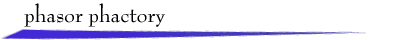A real, T-periodic signal  x(t)  can be represented by a Fourier series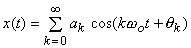where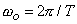is the fundamental frequency and each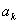is nonnegative (by adding p to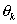if necessary).

This expression also can be written as the real part of a phasor sum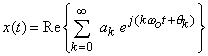For a gentle introduction to this topic, visit the lecture Harmonic Phasors and Fourier Series.

Since projection on the real (horizontal) axis is inconvenient for portrayal, we rotate each phasor by  p/2  radians and project on the vertical axis. This makes use of the mathematical relationship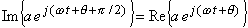In the applet there are four options for displaying a Fourier series with up to 25 terms:

·        select from the provided signals,

·        sketch one period of a signal,

·        construct a phasor sum by successively clicking on the complex plane,

·        enter data (the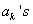, magnitude and angle) in a data table.

Amplitude units are marked on the complex plane, and the angles are in degrees.return to demonstrations page

 Applet by Hsi Chen Lee.